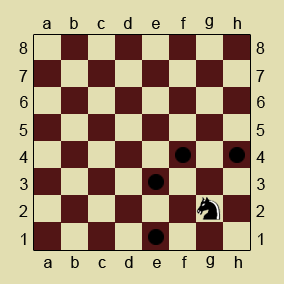# Knight Moves(CODEEVAL)

チェスのナイトの移動位置を求める問題。

```# 現在位置を(x, y)、次の移動候補を(a,b)とした場合
|a| + |b| = 3
1 <= x + a <= 8
1 <= y + b <= 8```

## CHALLENGE DESCRIPTION:

In chess, the knight moves to any of the closest squares that are not on the same rank, file, or diagonal. Thus the move is in the “L” form: two squares vertically and one square horizontally, or two squares horizontally and one square vertically:## INPUT SAMPLE:

The first argument is a filename that contains positions of the knight on the chessboard in the CN form, where:

1. C is a letter from “a” to “h” and denotes a column.
2. N is a number from 1 to 8 and denotes a row.

Each position is indicated in a new line.

```g2
a1
d6
e5
b1```

## OUTPUT SAMPLE:

```e1 e3 f4 h4
b3 c2
b5 b7 c4 c8 e4 e8 f5 f7
c4 c6 d3 d7 f3 f7 g4 g6
a3 c3 d2```

## CONSTRAINTS:

1. The number of test cases is 40.

## My Code

```#!/usr/bin/env ruby -w

module Chess
class Knight
# The codepoint of `a` is 97.
# The `a` is regarded as `1` in this program.
CORDINATE_DIFFERENCE_NUM = 96
def initialize
x = [1, -1].product [2, -2]
y = [2, -2].product [1, -1]
@moving_patterns = x + y
end

def candidates(x, y)
x = alphabet_to_cordinate(x)
y = y.to_i
@moving_patterns.inject([]) do |result, pattern|
a = x + pattern
b = y + pattern
result << "#{cordinate_to_alphabet(a)}#{b}" if include_board?(a) && include_board?(b)
result
end
end

private
def include_board?(n)
n >= 1 && n <= 8
end

def alphabet_to_cordinate(str)
str.ord - CORDINATE_DIFFERENCE_NUM
end

def cordinate_to_alphabet(x)
(x + CORDINATE_DIFFERENCE_NUM).chr
end
end
end

knight = Chess::Knight.new
ARGF.each_line do |line|
x, y = line.chomp.split("")
puts knight.candidates(x, y).sort.join(" ")
end
```